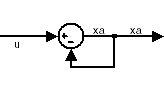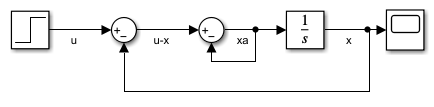## 代数环概念xa = u – xa.

### 数学解释

$\stackrel{˙}{x}=f\left(x,t\right),$

$\begin{array}{l}\stackrel{˙}{x}=f\left(x,{x}_{a},t\right)\\ 0=g\left(x,{x}_{a},t\right),\end{array}$

• fg 可以是向量函数。

• 第一个方程是微分方程。

• 第二个方程是代数方程。

• 微分变量的向量是 x。

• 代数变量的向量是 xax' = x

0 = u - x - 2xa

### 物理解释

• 在对物理系统建模时发生，通常是守恒定律所致，例如质量与能量守恒

• 当您为模型选择特定坐标系时发生

• 对动态系统中的系统响应的设计进行约束

### 人为代数环1. 将 Controller 和 Plant 模块包含在子系统中。

2. 在子系统对话框中，选择视为原子单元使子系统成为原子子系统。

3. 在模型配置参数的诊断窗格中，将代数环参数设置为 error### 代数环求解器的工作原理

Simulink 使用折线信赖域算法解算代数环。使用的容差小于 ODE 求解器 ReltolAbstol。这是因为 Simulink 使用“显式 ODE 方法”来解算指数为 1 的微分代数方程 (DAE)。

• 必须存在一个模块，在该模块中，代数环求解器可以中断循环并尝试解算该循环。

• 模型应该具有双精度实信号。

• 基础代数约束必须是一个平滑函数。xa(t) = u(t) /2. (1)

#### 代数环求解器中的信赖域算法和行搜索算法

• 信赖域

• 行搜索

set_param(model_name, 'AlgebraicLoopSolver','Auto');

set_param(model_name, 'AlgebraicLoopSolver', 'TrustRegion');

set_param(model_name, 'AlgebraicLoopSolver', 'LineSearch');

• Shampine 和 Reichelt 的 nleqn.m 代码

• User Guide for MINPACK-1（MINPACK-1 用户指南）中的 Fortran 程序 HYBRD12

• Numerical Methods for Nonlinear Algebraic Equations（非线性代数方程的数值方法）中 Powell 的“A Fortran subroutine for solving systems in nonlinear equations”（用于解算非线性系统的 Fortran 子例程）3

• 非线性最小化信赖域方法 (Optimization Toolbox).

• 线搜索 (Optimization Toolbox).

#### 代数环求解器的限制

• 带有离散值输出的模块

• 带有非双精度或复数输出的模块

• 不连续点

• Stateflow®

• 您不能为模型生成代码。